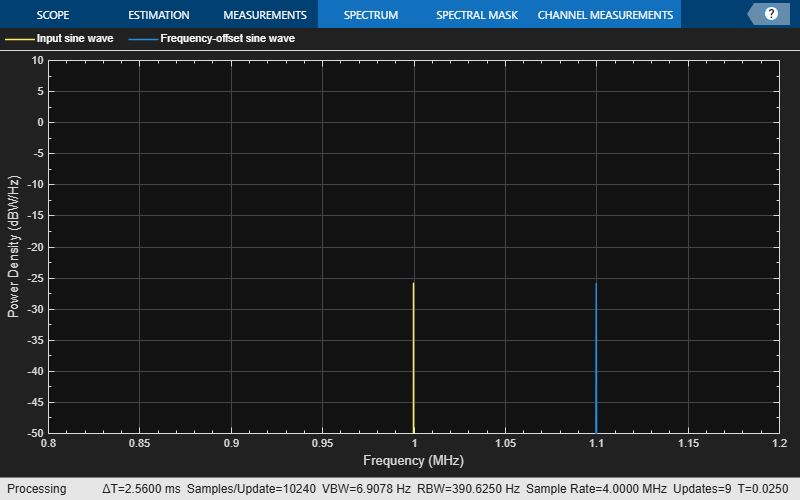# frequencyOffset

Apply frequency offset to input signal

Since R2022a

## Syntax

``y = frequencyOffset(x,samplerate,offset)``

## Description

example

````y = frequencyOffset(x,samplerate,offset)` applies the specified frequency offset to the input signal `x`.```

## Examples

collapse all

Generate a rectangular 16-point quadrature amplitude modulated (16-QAM) signal by modulating a vector of input data.

```M = 16; data = (0:M-1)'; x = qammod(data,M);```

Specify the signal sample rate and the frequency offset to apply.

```samplerate = 1; offset = 100e3;```

Apply the frequency offset to the input signal.

`y = frequencyOffset(x,samplerate,offset);`

Define parameters to configure the signal and spectrum analyzer.

```fc = 1e6; % Carrier frequency (Hz) fs = 4e6; % Sample rate (Hz) Nspf = 100e3; % Number of samples per frame freqSpan = 400e3; % Frequency span for spectrum computation (Hz)```

Create sine wave and spectrum analyzer objects with the specified parameter values.

```sinewave = dsp.SineWave(Amplitude=1, ... Frequency=fc, ... SampleRate=fs, ... SamplesPerFrame=Nspf, ... ComplexOutput=true); sascope = spectrumAnalyzer( ... SampleRate=fs, ... FrequencySpan="Span and center frequency", ... CenterFrequency=fc, ... Span=freqSpan, ... SpectrumType="Power density", ... SpectralAverages=10, ... SpectrumUnits="dBW/Hz", ... ShowLegend=true, ... ChannelNames=["Input sine wave","Frequency-offset sine wave"], ... YLimits=[-50 10]);```

Generate a sine wave signal.

`x = sinewave();`

Apply a frequency offset of 100 kHz to the signal.

```offset = 100e3; y = frequencyOffset(x,fs,offset);```

Display the input and frequency-shifted signals by using the spectrum analyzer.

`sascope(x,y)`## Input Arguments

collapse all

Input signal, specified as a column vector or matrix.

Data Types: `double` | `single`
Complex Number Support: Yes

Sampling rate of the input signal in Hz, specified as a positive scalar.

Data Types: `double`

Frequency offset in Hz, specified as a scalar or row vector.

• If `offset` is a scalar, the function applies the same frequency offset to each channel.

• If `offset` is a vector, then each element specifies the frequency offset that the function applies to the corresponding column (channel) of the input signal. The number of elements in `offset` must equal the number of columns in `x`.

Data Types: `double`

## Output Arguments

collapse all

Output signal, returned as a vector or matrix with the same dimensions and data type as `x`. The number of columns in `y` corresponds to the number of channels.

Data Types: `double` | `single`
Complex Number Support: Yes

## Version History

Introduced in R2022a